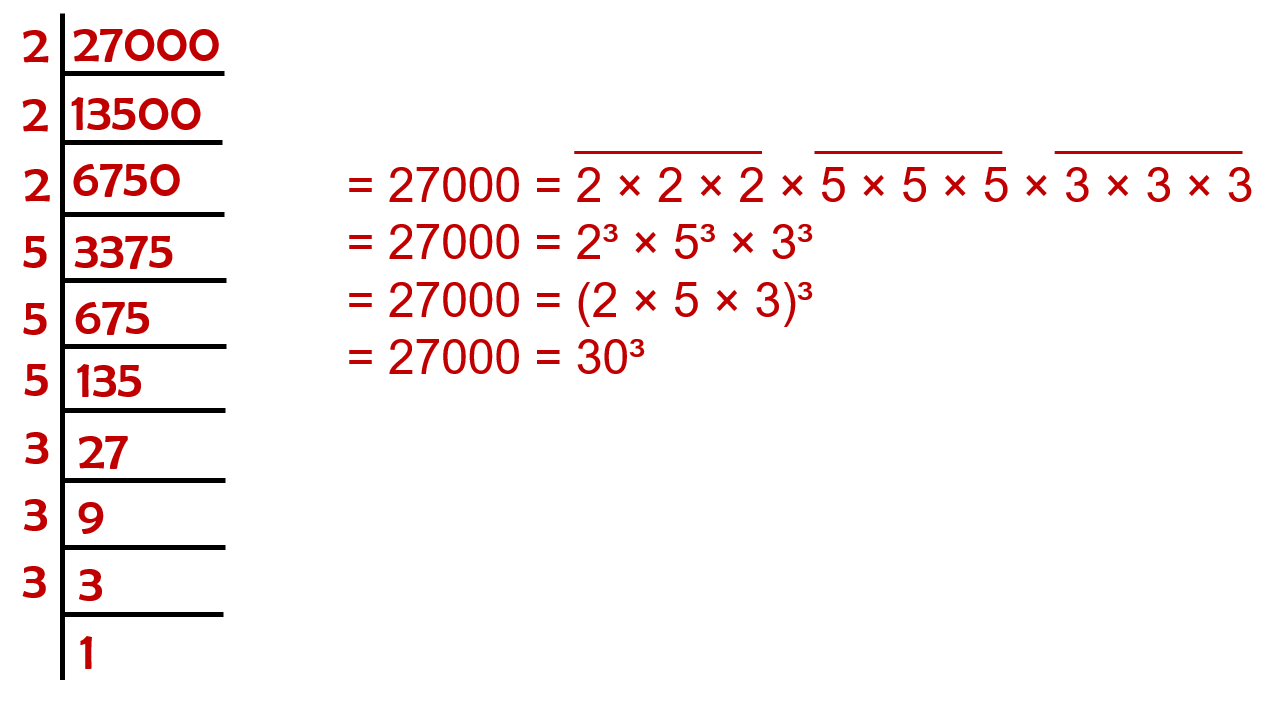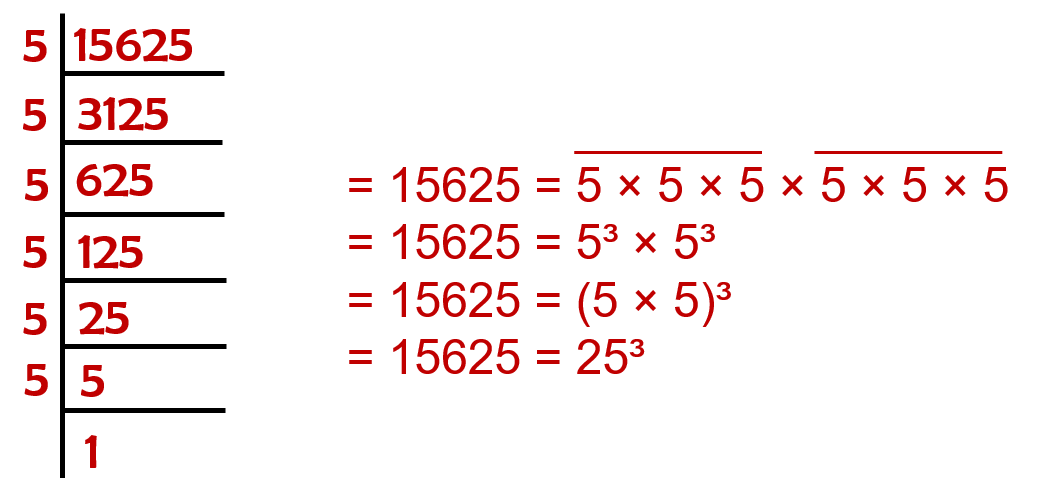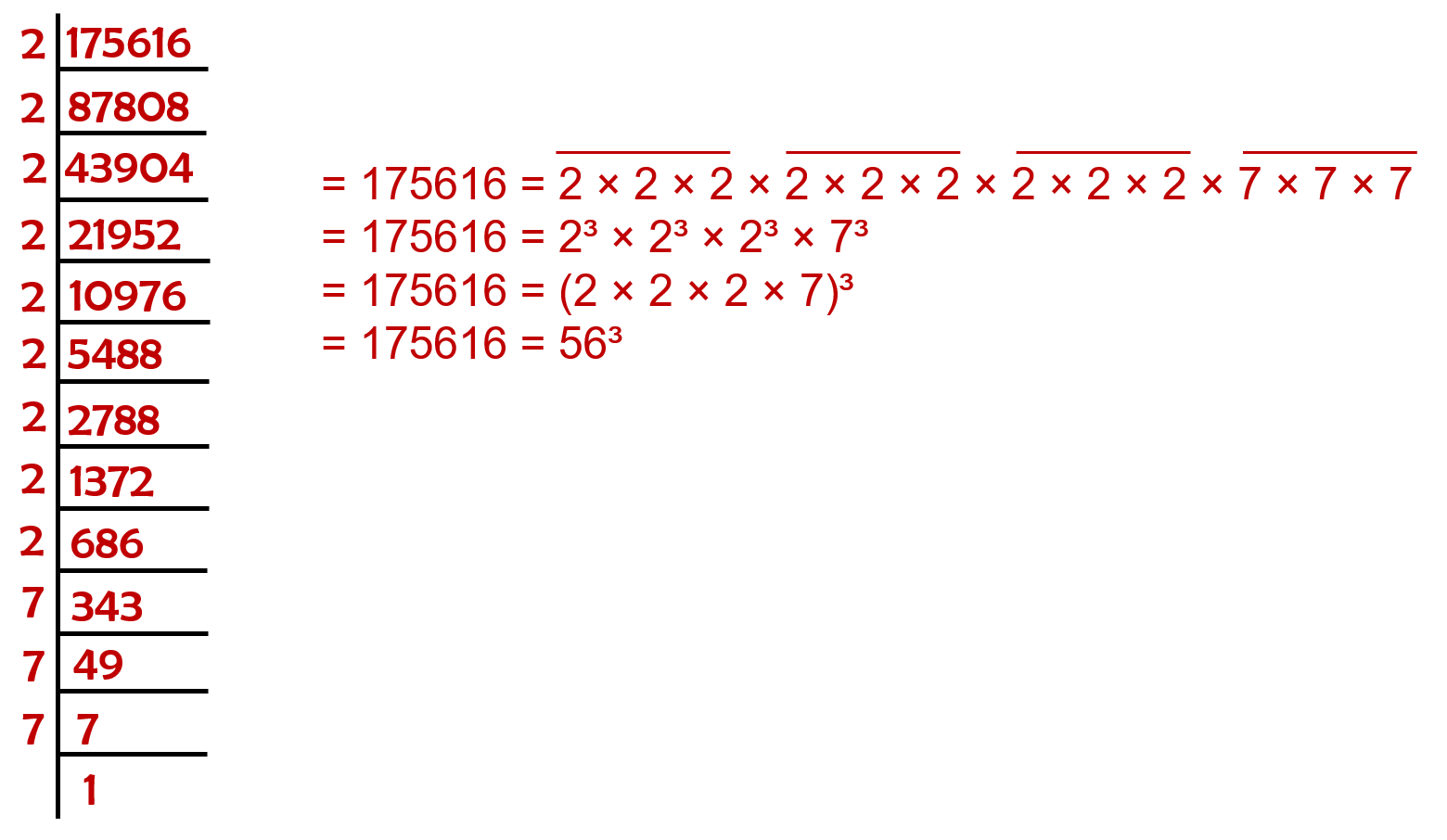# vs.eyeandcontacts.com

## Chapter 7 Cubes and Cube Roots Exercise 7.2

Question 1: Find the cube root of each of the following numbers by prime factorisation method.
(i) 64
(ii) 512
(iii) 10648
(iv) 27000
(v) 15625
(vi) 13824
(vii) 110592
(viii) 46656
(ix) 175616
(x) 91125

(i)Therefore, the cube root of 64 is 4.

(ii)Therefore, the cube root of 512 is 8.

(iii)Therefore, the cube root of 10648 is 22.

(iv)Therefore, the cube root of 27000 is 30.

(v)Therefore, the cube root of 15625 is 25.

(vi)Therefore, the cube root of 13824.

(vii)Therefore, the cube root of 110592 is 48.

(viii)Therefore, the cube root of 46656 is 36.

(ix)Therefore, the cube root of 175616 is 56.

(x)Therefore, the cube root of 91125 is 45.

Question 2: State true or false.
(i) Cube of any odd number is even.
(ii) A perfect cube does not end with two zeros.
(iii) If square of a number ends with 5, then its cube ends with 25.
(iv) There is no perfect cube which ends with 8.
(v) The cube of a two digit number may be a three digit number.
(vi) The cube of a two digit number may have seven or more digits.
(vii) The cube of a single digit number may be a single digit number.

(i) False
(ii) True
(iii) False
(iv) False
(v) False
(vi) False
(vii) True

Question 3: You are told that 1,331 is a perfect cube. Can you guess without factorisation what is its cube root? Similarly, guess the cube roots of 4913, 12167, 32768.

• 1331
Form groups of three starting from the rightmost digit of 1331.
1 3 3 1
Take 331. If the number ends with 1, its unit digit of cube root will be 1.
Take 1 (other group).
= 1³ = 1
= 2³ = 8
= 1³ = 1 < 2³
The one’s place of 1 is 1 itself. Take 1 as ten’s place of the cube root of 1331.
Therefore, ∛1331 = 11

• 4913
Form groups of three starting from the rightmost digit of 1331.
4 9 1 3
Take 913. If the number ends with 3, its unit digit of cube root will be 7.
Take 4 (other group).
= 1³ = 1
= 2³ = 8
= 1³ < 4 < 2³
The one’s place of 1 is 1 itself. Take 1 as ten’s place of the cube root of 4913.
Therefore, ∛4913 = 17

• 12167
Form groups of three starting from the rightmost digit of 1331.
1 2 1 6 7
Take 167. If the number ends with 7, its unit digit of cube root will be 3.
Take 12 (other group).
= 2³ = 8
= 3³ = 27
= 2³ < 12 < 3³
The one’s place of 2 is 2 itself. Take 2 as ten’s place of the cube root of 12167.
Therefore, ∛12167 = 22.

• 32768
Form groups of three starting from the rightmost digit of 32768.
3 2 7 6 8
Take 768. If the number ends with 8, its unit digit of cube root will be 2.
Take 32 (other group).
= 3³ = 27
= 4³ = 64
= 3³ < 32 < 4³
The one’s place of 3 is 3 itself. Take 3 as ten’s place of the cube root of 32768.
Therefore, ∛32768 = 32.